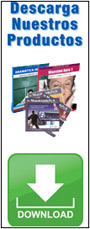>Números

 · 1 = One · 2 = Two · 3 = Three · 4 = Four · 5 = Five · 6 = Six · 7 = Seven · 8 = Eight · 9 = Nine · 10 = Ten· 11 = Eleven · 12 = Twelve · 13 = Thirteen · 14 = Fourteen · 15 = Fifteen · 16 = Sixteen · 17 = Seventeen · 18 = Eighteen · 19 = Nineteen · 20 = Twenty · 30 = Thirty · 40 = Forty · 50 = Fifty · 60 = Sixty · 70 = Seventy · 80 = Eighty · 90 = Ninety · 21 = Twenty-one · 100 = One hundred · 101 = One hundred and one · 1000 = One thousand · 2510 = Two thousand, five hundred and ten · 1000000 = One million· En inglés se usa la coma como separador de miles y el punto como separador decimal. - 2,000 = Two thousand / 2.95 = Two point nine five · El número cero ('0') se pronuncia como "zero" o como "o" (como la letra 'o').

Más detalle en nuestra Gramática Inglesa Gratis
La Mansión del Inglés - www.mansioningles.comDescargar / Imprimir FichaAcceder a todas las fichas

TAMBIÉN TE PUEDE INTERESAR:

La Mansión del Inglés. https://www.mansioningles.com